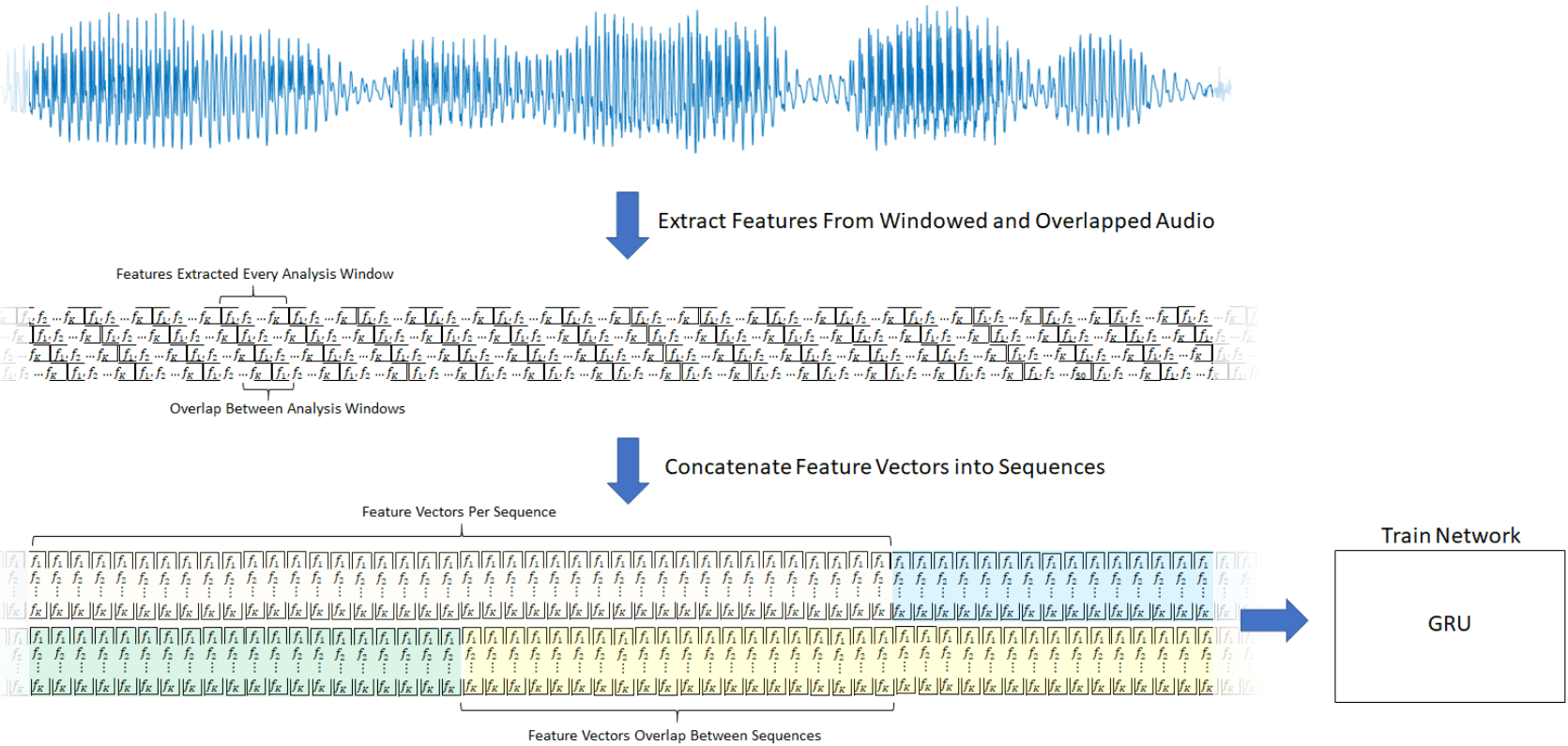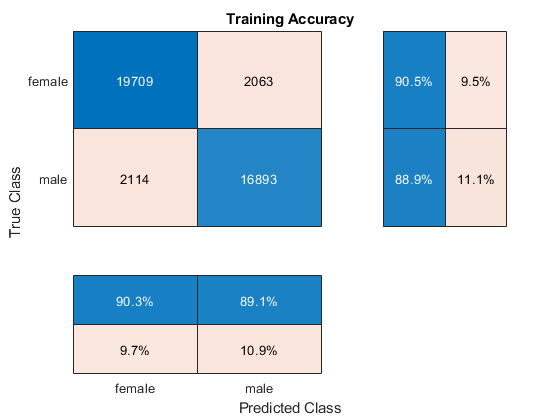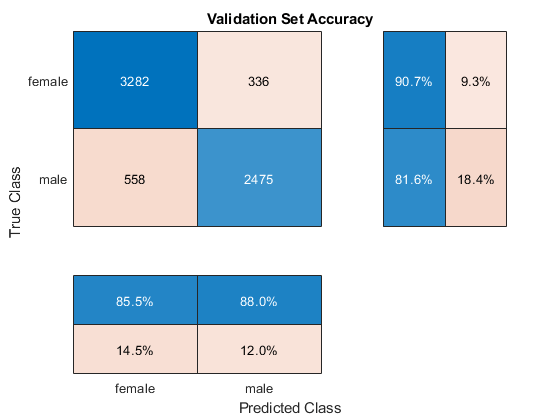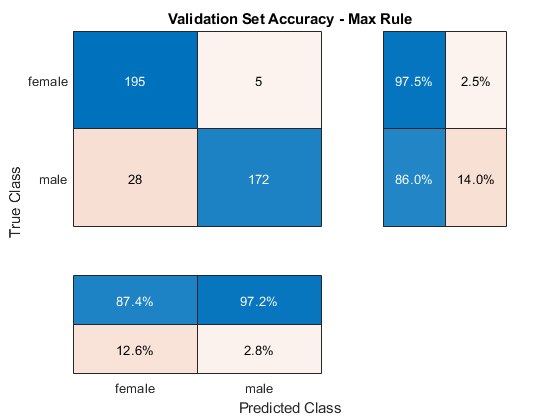# Classify Gender Using LSTM Networks

This example shows how to classify the gender of a speaker using deep learning. The example uses a Bidirectional Long Short-Term Memory (BiLSTM) network and Gammatone Cepstral Coefficients (gtcc), pitch, harmonic ratio, and several spectral shape descriptors.

### Introduction

Gender classification based on speech signals is an essential component of many audio systems, such as automatic speech recognition, speaker recognition, and content-based multimedia indexing.

This example uses long short-term memory (LSTM) networks, a type of recurrent neural network (RNN) well-suited to study sequence and time-series data. An LSTM network can learn long-term dependencies between time steps of a sequence. An LSTM layer (`lstmLayer` (Deep Learning Toolbox)) can look at the time sequence in the forward direction, while a bidirectional LSTM layer (`bilstmLayer` (Deep Learning Toolbox)) can look at the time sequence in both forward and backward directions. This example uses bidirectional LSTM layers.

This example trains the LSTM network with sequences of gammatone cepstrum coefficients (`gtcc`), pitch estimates (`pitch`), harmonic ratio (`harmonicRatio`), and several spectral shape descriptors (Spectral Descriptors).

To accelerate the training process, run this example on a machine with a GPU. If your machine has a GPU and Parallel Computing Toolbox™, then MATLAB© automatically uses the GPU for training; otherwise, it uses the CPU.

### Classify Gender with a Pre-Trained Network

Before going into the training process in detail, you will use a pre-trained network to classify the gender of the speaker in two test signals.

Load the pre-trained network along with pre-computed vectors used for feature normalization.

`load('genderIDNet.mat', 'genderIDNet', 'M', 'S');`

Load a test signal with a male speaker.

```[audioIn, Fs] = audioread('maleSpeech.flac'); sound(audioIn, Fs)```

Isolate the speech area in the signal.

```boundaries = detectSpeech(audioIn, Fs); audioIn = audioIn(boundaries(1):boundaries(2));```

Create an `audioFeatureExtractor` to extract features from the audio data. You will use the same object to extract features for training.

```extractor = audioFeatureExtractor( ... "SampleRate",Fs, ... "Window",hamming(round(0.03*Fs),"periodic"), ... "OverlapLength",round(0.02*Fs), ... ... "gtcc",true, ... "gtccDelta",true, ... "gtccDeltaDelta",true, ... ... "SpectralDescriptorInput","melSpectrum", ... "spectralCentroid",true, ... "spectralEntropy",true, ... "spectralFlux",true, ... "spectralSlope",true, ... ... "pitch",true, ... "harmonicRatio",true);```

Extract features from the signal and normalize them.

```features = extract(extractor, audioIn); features = (features.' - M)./S;```

Classify the signal.

`gender = classify(genderIDNet, features)`
```gender = categorical male ```

Classify another signal with a female speaker.

```[audioIn, Fs] = audioread('femaleSpeech.flac'); sound(audioIn, Fs)```
```boundaries = detectSpeech(audioIn, Fs); audioIn = audioIn(boundaries(1):boundaries(2)); features = extract(extractor, audioIn); features = (features.' - M)./S; classify(genderIDNet, features)```
```ans = categorical female ```

### Preprocess Training Audio Data

The BiLSTM network used in this example works best when using sequences of feature vectors. To illustrate the preprocessing pipeline, this example walks through the steps for a single audio file.

Read the contents of an audio file containing speech. The speaker gender is male.

```[audioIn,Fs] = audioread('Counting-16-44p1-mono-15secs.wav'); labels = {'male'};```

Plot the audio signal and then listen to it using the `sound` command.

```timeVector = (1/Fs) * (0:size(audioIn,1)-1); figure plot(timeVector,audioIn) ylabel("Amplitude") xlabel("Time (s)") title("Sample Audio") grid on````sound(audioIn,Fs)`

The speech signal has silence segments that do not contain useful information pertaining to the gender of the speaker. Use `detectSpeech` to locate segments of speech in the audio signal.

`speechIndices = detectSpeech(audioIn,Fs);`

Create an `audioFeatureExtractor` to extract features from the audio data. A speech signal is dynamic in nature and changes over time. It is assumed that speech signals are stationary on short time scales and their processing is often done in windows of 20-40 ms. Specify 30 ms windows with 20 ms overlap.

```extractor = audioFeatureExtractor( ... "SampleRate",Fs, ... "Window",hamming(round(0.03*Fs),"periodic"), ... "OverlapLength",round(0.02*Fs), ... ... "gtcc",true, ... "gtccDelta",true, ... "gtccDeltaDelta",true, ... ... "SpectralDescriptorInput","melSpectrum", ... "spectralCentroid",true, ... "spectralEntropy",true, ... "spectralFlux",true, ... "spectralSlope",true, ... ... "pitch",true, ... "harmonicRatio",true);```

Extract features from each audio segment. The output from `audioFeatureExtractor` is a `numFeatureVectors`-by-`numFeatures` array. The `sequenceInputLayer` used in this example requires time to be along the second dimension. Permute the output array so that time is along the second dimension.

```featureVectorsSegment = {}; for ii = 1:size(speechIndices,1) featureVectorsSegment{end+1} = ( extract(extractor,audioIn(speechIndices(ii,1):speechIndices(ii,2))) )'; end numSegments = size(featureVectorsSegment)```
```numSegments = 1×2 1 11 ```
`[numFeatures,numFeatureVectorsSegment1] = size(featureVectorsSegment{1})`
```numFeatures = 45 ```
```numFeatureVectorsSegment1 = 124 ```

Replicate the labels so that they are in one-to-one correspondence with segments.

`labels = repelem(labels,size(speechIndices,1))`
```labels = 1×11 cell {'male'} {'male'} {'male'} {'male'} {'male'} {'male'} {'male'} {'male'} {'male'} {'male'} {'male'} ```

When using a `sequenceInputLayer`, it is often advantageous to use sequences of consistent length. Convert the arrays of feature vectors into sequences of feature vectors. Use 20 feature vectors per sequence with 5 feature vector overlap.

```featureVectorsPerSequence = 20; featureVectorOverlap = 5; hopLength = featureVectorsPerSequence - featureVectorOverlap; idx1 = 1; featuresTrain = {}; sequencePerSegment = zeros(numel(featureVectorsSegment),1); for ii = 1:numel(featureVectorsSegment) sequencePerSegment(ii) = max(floor((size(featureVectorsSegment{ii},2) - featureVectorsPerSequence)/hopLength) + 1,0); idx2 = 1; for j = 1:sequencePerSegment(ii) featuresTrain{idx1,1} = featureVectorsSegment{ii}(:,idx2:idx2 + featureVectorsPerSequence - 1); idx1 = idx1 + 1; idx2 = idx2 + hopLength; end end```

For conciseness, the helper function HelperFeatureVector2Sequence encapsulates the above processing and is used throughout the rest of the example.

Replicate the labels so that they are in one-to-one correspondence with the training set.

`labels = repelem(labels,sequencePerSegment);`

The result of the preprocessing pipeline is a `NumSequence`-by-1 cell array of `NumFeatures`-by-`FeatureVectorsPerSequence` matrices. Labels is a `NumSequence`-by-1 array.

`NumSequence = numel(featuresTrain)`
```NumSequence = 27 ```
`[NumFeatures,FeatureVectorsPerSequence] = size(featuresTrain{1})`
```NumFeatures = 45 ```
```FeatureVectorsPerSequence = 20 ```
`NumSequence = numel(labels)`
```NumSequence = 27 ```

The figure provides an overview of the feature extraction used per detected speech region.### Create Training and Test Datastores

This example uses a subset of the Mozilla Common Voice dataset . The dataset contains 48 kHz recordings of subjects speaking short sentences. Download the dataset and untar the downloaded file. Set `PathToDatabase` to the location of the data.

```url = 'http://ssd.mathworks.com/supportfiles/audio/commonvoice.zip'; downloadFolder = tempdir; dataFolder = fullfile(downloadFolder,'commonvoice'); if ~exist(dataFolder,'dir') disp('Downloading data set (956 MB) ...') unzip(url,downloadFolder) end```

Use `audioDatastore` to create datastores for the training and validation sets. Use `readtable` to read the metadata associated with the audio files.

```loc = fullfile(dataFolder); adsTrain = audioDatastore(fullfile(loc,'train'),'IncludeSubfolders',true); metadataTrain = readtable(fullfile(fullfile(loc,'train'),"train.tsv"),"FileType","text"); adsTrain.Labels = metadataTrain.gender; adsValidation = audioDatastore(fullfile(loc,'validation'),'IncludeSubfolders',true); metadataValidation = readtable(fullfile(fullfile(loc,'validation'),"validation.tsv"),"FileType","text"); adsValidation.Labels = metadataValidation.gender;```

Use `countEachLabel` to inspect the gender breakdown of the training and validation sets.

`countEachLabel(adsTrain)`
```ans=2×2 table Label Count ______ _____ female 1000 male 1000 ```
`countEachLabel(adsValidation)`
```ans=2×2 table Label Count ______ _____ female 200 male 200 ```

To train the network with the entire dataset and achieve the highest possible accuracy, set `reduceDataset` to `false`. To run this example quickly, set `reduceDataset` to `true`.

```reduceDataset = false; if reduceDataset % Reduce the training dataset by a factor of 20 adsTrain = splitEachLabel(adsTrain,round(numel(adsTrain.Files) / 2 / 20)); adsValidation = splitEachLabel(adsValidation,20); end```

### Create Training and Validation Sets

Determine the sample rate of audio files in the data set, and then update the sample rate, window, and overlap length of the audio feature extractor.

```[~,adsInfo] = read(adsTrain); Fs = adsInfo.SampleRate; extractor.SampleRate = Fs; extractor.Window = hamming(round(0.03*Fs),"periodic"); extractor.OverlapLength = round(0.02*Fs);```

To speed up processing, distribute computations over multiple workers. If you have Parallel Computing Toolbox™, the example partitions the datastore so that the feature extraction occurs in parallel across available workers. Determine the optimal number of partitions for your system. If you do not have Parallel Computing Toolbox™, the example uses a single worker.

```if ~isempty(ver('parallel')) && ~reduceDataset pool = gcp; numPar = numpartitions(adsTrain,pool); else numPar = 1; end```

In a loop:

1. Read from the audio datastore.

2. Detect regions of speech.

3. Extract feature vectors from the regions of speech.

Replicate the labels so that they are in one-to-one correspondence with the feature vectors.

```labelsTrain = []; featureVectors = {}; % Loop over optimal number of partitions parfor ii = 1:numPar % Partition datastore subds = partition(adsTrain,numPar,ii); % Preallocation featureVectorsInSubDS = {}; segmentsPerFile = zeros(numel(subds.Files),1); % Loop over files in partitioned datastore for jj = 1:numel(subds.Files) % 1. Read in a single audio file audioIn = read(subds); % 2. Determine the regions of the audio that correspond to speech speechIndices = detectSpeech(audioIn,Fs); % 3. Extract features from each speech segment segmentsPerFile(jj) = size(speechIndices,1); features = cell(segmentsPerFile(jj),1); for kk = 1:size(speechIndices,1) features{kk} = ( extract(extractor,audioIn(speechIndices(kk,1):speechIndices(kk,2))) )'; end featureVectorsInSubDS = [featureVectorsInSubDS;features(:)]; end featureVectors = [featureVectors;featureVectorsInSubDS]; % Replicate the labels so that they are in one-to-one correspondance % with the feature vectors. repedLabels = repelem(subds.Labels,segmentsPerFile); labelsTrain = [labelsTrain;repedLabels(:)]; end```

In classification applications, it is good practice to normalize all features to have zero mean and unity standard deviation.

Compute the mean and standard deviation for each coefficient, and use them to normalize the data.

```allFeatures = cat(2,featureVectors{:}); allFeatures(isinf(allFeatures)) = nan; M = mean(allFeatures,2,'omitnan'); S = std(allFeatures,0,2,'omitnan'); featureVectors = cellfun(@(x)(x-M)./S,featureVectors,'UniformOutput',false); for ii = 1:numel(featureVectors) idx = find(isnan(featureVectors{ii})); if ~isempty(idx) featureVectors{ii}(idx) = 0; end end```

Buffer the feature vectors into sequences of 20 feature vectors with 10 overlap. If a sequence has less than 20 feature vectors, drop it.

`[featuresTrain,trainSequencePerSegment] = HelperFeatureVector2Sequence(featureVectors,featureVectorsPerSequence,featureVectorOverlap);`

Replicate the labels so that they are in one-to-one correspondence with the sequences.

```labelsTrain = repelem(labelsTrain,[trainSequencePerSegment{:}]); labelsTrain = categorical(labelsTrain);```

Create the validation set using the same steps used to create the training set.

```labelsValidation = []; featureVectors = {}; valSegmentsPerFile = []; parfor ii = 1:numPar subds = partition(adsValidation,numPar,ii); featureVectorsInSubDS = {}; valSegmentsPerFileInSubDS = zeros(numel(subds.Files),1); for jj = 1:numel(subds.Files) audioIn = read(subds); speechIndices = detectSpeech(audioIn,Fs); numSegments = size(speechIndices,1); features = cell(valSegmentsPerFileInSubDS(jj),1); for kk = 1:numSegments features{kk} = ( extract(extractor,audioIn(speechIndices(kk,1):speechIndices(kk,2))) )'; end featureVectorsInSubDS = [featureVectorsInSubDS;features(:)]; valSegmentsPerFileInSubDS(jj) = numSegments; end repedLabels = repelem(subds.Labels,valSegmentsPerFileInSubDS); labelsValidation = [labelsValidation;repedLabels(:)]; featureVectors = [featureVectors;featureVectorsInSubDS]; valSegmentsPerFile = [valSegmentsPerFile;valSegmentsPerFileInSubDS]; end featureVectors = cellfun(@(x)(x-M)./S,featureVectors,'UniformOutput',false); for ii = 1:numel(featureVectors) idx = find(isnan(featureVectors{ii})); if ~isempty(idx) featureVectors{ii}(idx) = 0; end end [featuresValidation,valSequencePerSegment] = HelperFeatureVector2Sequence(featureVectors,featureVectorsPerSequence,featureVectorOverlap); labelsValidation = repelem(labelsValidation,[valSequencePerSegment{:}]); labelsValidation = categorical(labelsValidation);```

### Define the LSTM Network Architecture

LSTM networks can learn long-term dependencies between time steps of sequence data. This example uses the bidirectional LSTM layer `bilstmLayer` to look at the sequence in both forward and backward directions.

Specify the input size to be sequences of size `NumFeatures`. Specify a hidden bidirectional LSTM layer with an output size of 50 and output a sequence. Then, specify a bidirectional LSTM layer with an output size of 50 and output the last element of the sequence. This command instructs the bidirectional LSTM layer to map its input into 50 features and then prepares the output for the fully connected layer. Finally, specify two classes by including a fully connected layer of size 2, followed by a softmax layer and a classification layer.

```layers = [ ... sequenceInputLayer(size(featuresTrain{1},1)) bilstmLayer(50,"OutputMode","sequence") bilstmLayer(50,"OutputMode","last") fullyConnectedLayer(2) softmaxLayer classificationLayer];```

Next, specify the training options for the classifier. Set `MaxEpochs` to `4` so that the network makes 4 passes through the training data. Set `MiniBatchSize` of 256 so that the network looks at 128 training signals at a time. Specify `Plots` as `"training-progress"` to generate plots that show the training progress as the number of iterations increases. Set `Verbose` to `false` to disable printing the table output that corresponds to the data shown in the plot. Specify `Shuffle` as `"every-epoch"` to shuffle the training sequence at the beginning of each epoch. Specify `LearnRateSchedule` to `"piecewise"` to decrease the learning rate by a specified factor (0.1) every time a certain number of epochs (1) has passed.

This example uses the adaptive moment estimation (ADAM) solver. ADAM performs better with recurrent neural networks (RNNs) like LSTMs than the default stochastic gradient descent with momentum (SGDM) solver.

```miniBatchSize = 256; validationFrequency = floor(numel(labelsTrain)/miniBatchSize); options = trainingOptions("adam", ... "MaxEpochs",4, ... "MiniBatchSize",miniBatchSize, ... "Plots","training-progress", ... "Verbose",false, ... "Shuffle","every-epoch", ... "LearnRateSchedule","piecewise", ... "LearnRateDropFactor",0.1, ... "LearnRateDropPeriod",1, ... 'ValidationData',{featuresValidation,labelsValidation}, ... 'ValidationFrequency',validationFrequency);```

### Train the LSTM Network

Train the LSTM network with the specified training options and layer architecture using `trainNetwork`. Because the training set is large, the training process can take several minutes.

`net = trainNetwork(featuresTrain,labelsTrain,layers,options);`The top subplot of the training-progress plot represents the training accuracy, which is the classification accuracy on each mini-batch. When training progresses successfully, this value typically increases towards 100%. The bottom subplot displays the training loss, which is the cross-entropy loss on each mini-batch. When training progresses successfully, this value typically decreases towards zero.

If the training is not converging, the plots might oscillate between values without trending in a certain upward or downward direction. This oscillation means that the training accuracy is not improving and the training loss is not decreasing. This situation can occur at the start of training, or after some preliminary improvement in training accuracy. In many cases, changing the training options can help the network achieve convergence. Decreasing `MiniBatchSize` or decreasing `InitialLearnRate` might result in a longer training time, but it can help the network learn better.

### Visualize the Training Accuracy

Calculate the training accuracy, which represents the accuracy of the classifier on the signals on which it was trained. First, classify the training data.

`prediction = classify(net,featuresTrain);`

Plot the confusion matrix. Display the precision and recall for the two classes by using column and row summaries.

```figure cm = confusionchart(categorical(labelsTrain),prediction,'title','Training Accuracy'); cm.ColumnSummary = 'column-normalized'; cm.RowSummary = 'row-normalized';```### Visualize the Validation Accuracy

Calculate the validation accuracy. First, classify the training data.

`[prediction,probabilities] = classify(net,featuresValidation);`

Plot the confusion matrix. Display the precision and recall for the two classes by using column and row summaries.

```figure cm = confusionchart(categorical(labelsValidation),prediction,'title','Validation Set Accuracy'); cm.ColumnSummary = 'column-normalized'; cm.RowSummary = 'row-normalized';```The example generated multiple sequences from each training speech file. Higher accuracy can be achieved by considering the output class of all sequences corresponding to the same file, and applying a "max-rule" decision, where the class with the segment with the highest confidence score is selected.

Determine the number of sequences generated per file in the validation set.

```sequencePerFile = zeros(size(valSegmentsPerFile)); valSequencePerSegmentMat = cell2mat(valSequencePerSegment); idx = 1; for ii = 1:numel(valSegmentsPerFile) sequencePerFile(ii) = sum(valSequencePerSegmentMat(idx:idx+valSegmentsPerFile(ii)-1)); idx = idx + valSegmentsPerFile(ii); end```

Predict the gender from each training file by considering the output classes of all sequences generated from the same file.

```numFiles = numel(adsValidation.Files); actualGender = categorical(adsValidation.Labels); predictedGender = actualGender; scores = cell(1,numFiles); counter = 1; cats = unique(actualGender); for index = 1:numFiles scores{index} = probabilities(counter: counter + sequencePerFile(index) - 1,:); m = max(mean(scores{index},1),[],1); if m(1) >= m(2) predictedGender(index) = cats(1); else predictedGender(index) = cats(2); end counter = counter + sequencePerFile(index); end```

Visualize the confusion matrix on the majority-rule predictions.

```figure cm = confusionchart(actualGender,predictedGender,'title','Validation Set Accuracy - Max Rule'); cm.ColumnSummary = 'column-normalized'; cm.RowSummary = 'row-normalized';```### Supporting Functions

```function [sequences,sequencePerSegment] = HelperFeatureVector2Sequence(features,featureVectorsPerSequence,featureVectorOverlap) if featureVectorsPerSequence <= featureVectorOverlap error('The number of overlapping feature vectors must be less than the number of feature vectors per sequence.') end hopLength = featureVectorsPerSequence - featureVectorOverlap; idx1 = 1; sequences = {}; sequencePerSegment = cell(numel(features),1); for ii = 1:numel(features) sequencePerSegment{ii} = max(floor((size(features{ii},2) - featureVectorsPerSequence)/hopLength) + 1,0); idx2 = 1; for j = 1:sequencePerSegment{ii} sequences{idx1,1} = features{ii}(:,idx2:idx2 + featureVectorsPerSequence - 1); %#ok<AGROW> idx1 = idx1 + 1; idx2 = idx2 + hopLength; end end end```

## SupportGet trial now